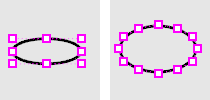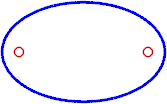# EllipseCurve

Ellipse >

<Options>

The Ellipse command draws a closed elliptical curve.

#### Steps

1. Pick the center.
2. Pick the end of the first axis.
3. Pick the end of second axis.
Command-line options
##### Default

Creates the ellipse from the center, first axis, and second axis.

#### Default steps

1. Pick the center.
2. Pick the end of the first axis.
3. Pick the end of second axis.

##### Deformable

A NURBS approximation of the curve will be created with the degree and number of points you specify.Not deformable degree 2 (left); Deformable degree 3 (right).
##### PointCount

Sets the number of control points in the curve.

##### Vertical

Draws the ellipse center point and axes perpendicular to the construction plane.

#### Vertical steps

1. Pick the center.
2. Pick end of the first axis.
3. Pick the end of the second axis.

##### Corner

Draws the ellipse from the corners of an enclosing rectangle.

#### Corner steps

1. Pick a corner for the enclosing rectangle.
2. Pick the opposite corner of the enclosing rectangle.

##### Diameter

Draws the ellipse from points on its axes.

#### Diameter steps

1. Pick the start of the first axis.
2. Pick the end of the first axis.
3. Pick the end of the second axis.
##### FromFoci

Draws the ellipse from focus points and a point on the curve.

#### FromFoci steps

1. Pick the first focus.
2. Pick the second focus.
3. Pick a point on the ellipse curve.
##### MarkFoci

Places a point object at the focus locations.##### AroundCurve

Draws the ellipse around a curve.

#### Steps

1. Pick the center on a curve.
2. Pick the end of the first axis.
3. Pick the end of the second axis.